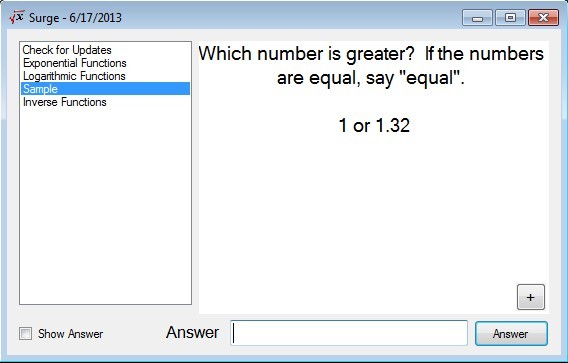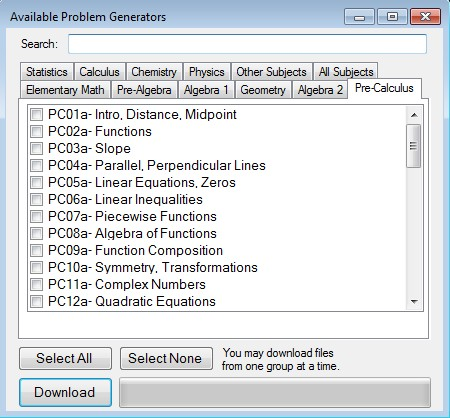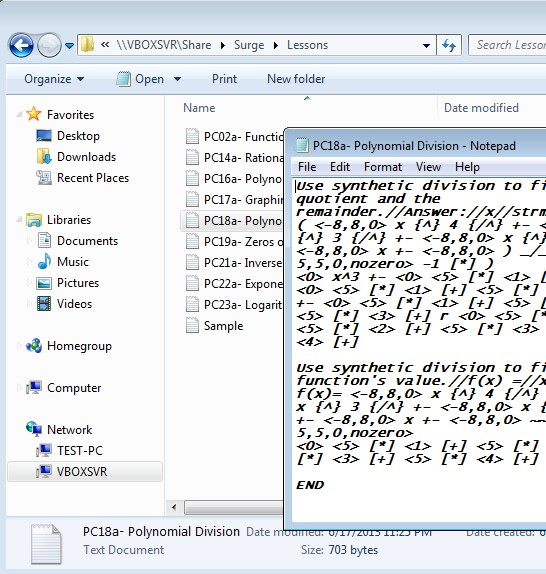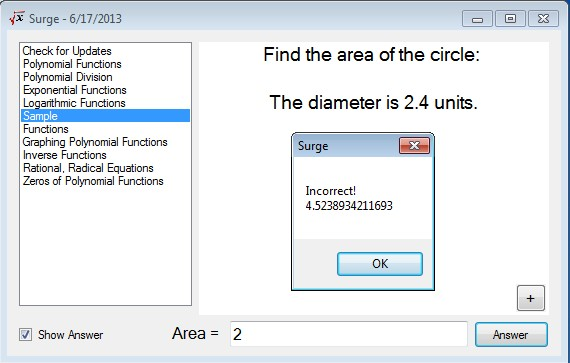Editor Ratings:
User Ratings:
[Total: 0 Average: 0]

Surge is a free mathematical problem generator for Windows which you can use to randomly create a large number of different mathematical problems ranging from basic math like multiplication tables, additions, number comparisons to more advanced stuff like quadratic equations, functions, trigonometry and so on. Every problem that’s created also requires you to type in a solution and that way you can see if what you’ve calculated is correct.

Example of a mathematical problem which was generated with Surge can be seen on the image below. This particular problem was generated using the simple category of Surge’s mathematical problem generator, where obviously you only get simple problems like the number comparison that’s shown.## Key features of Surge: free mathematical problem generator for Windows are:

• Portable: works without installation, just click and run to use
• Quickly generates random mathematical problems of varying difficulty
• Supports simple math, functions, equations, geometry, complex#, etc
• Expandable functionality with additional problem types using files
• Allows you to see the answer in case you didn’t answer correctly

Similar software: Bubble Slums.

After running Surge for the first time, the only type of mathematical problems that you’ll get are simple math equations. Additional categories that we mentioned, several dozens of them, will have to be downloaded additionally from the web. They’re gonna be downloaded in a separate folder called Lessons as text documents. Problem categories can be edited manually so that you yourself can add additional problem variations to the existing ones.

## How to generate math problems with Surge:By clicking on the plus button in the bottom right corner of the interface you can open up the selector from where you can download additional problem categories, functions, equations and everything else that we mentioned. Make a selection by putting check marks next to category and then click download button down below to start the download.Example of how a math problem setup in the downloaded text file looks like can be seen on the screenshot above. Setup syntax needs to be followed if you want to edit existing problems, if you want to edit variations that they put out.Alternatively, you can just start using the math problem generator to start creating and solving created problems. If you need help solving the problem you can put a check mark next to the Show Answer option. Solution to the generated problem needs to be typed in in the field all the way down below. Use the left sidebar to switch between the problem categories that you’ve just downloaded from the plus button menu.

## Conclusion

Surge is a great way how you can easily generate math problems for you if you’re practicing math or if you’re tutoring someone and you need a lot of math problems to give to your student. It’s portable, which means that if you’re only interested in testing out and seeing how this free mathematical problem generator works you don’t have to go through installation. On top of all that it’s very easy to use and offers a large selection of math problem type.